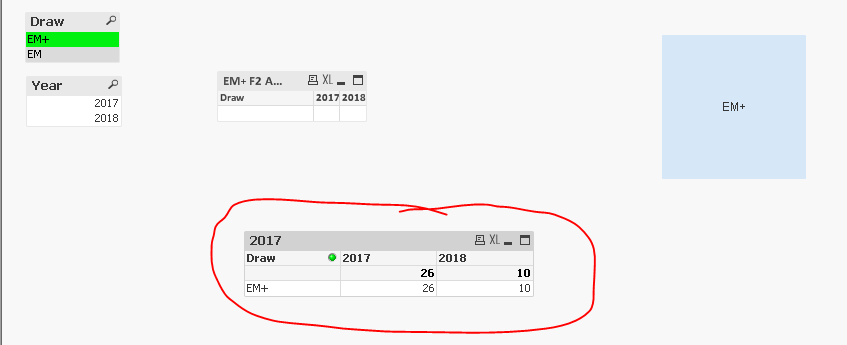# New to Qlik Sense

Discussion board where members can get started with Qlik Sense.

Contributor II

## Aggr Set Analysis Calculated Dimension with Variable

Hi All,

I have created an expression in Calculated Dimension but its not showing the result. Can you please advise me where I am having an issue. If I removed the ( Draw={"=\$(=Draw_EM)"} ) this part then its work but when I restrict by field selection Draw then its showing blank.

I have attached the qvw file.

Draw_EM is a Variable:

=if(GetSelectedCount(Draw) > 0 , GetFieldSelections(Draw), 'EM+')

Calculated Dimension:

=Only({\$<Draw={"=\$(=Draw_EM)"}, Period={">=\$(=vPeriod_EM)"}>} Draw)

Expression:

=Count({\$<Draw={"=\$(=Draw_EM)"}, Draw = {"=\$(=Draw_EM)"}, Year = {"2017"}>} F2)

Kind regards,

Farrukh

1 Solution

Accepted SolutionsMVP

## Re: Aggr Set Analysis Calculated Dimension with Variable

Got it... check attached

9 RepliesMVP

## Re: Aggr Set Analysis Calculated Dimension with Variable

How about using this

=If(GetSelectedCount(Draw) = 0, Count({\$<Draw={'EM+'}, Year = {"2017"}>} F2), Count({\$<Year = {"2017"}>} F2))Contributor II

## Re: Aggr Set Analysis Calculated Dimension with Variable

Hi Sunny,

Sorry for replying late, its a good idea for dimension but I have apx 50 expressions. its mean I need to apply IF statement in each column expression.

Can you please advise me without the IF Statement? I want to use \$(Variable) instead of IF statement.

Calculated Dimension:

=Aggr(Only({\$<Draw={"=\$(=Draw_EM)"}, Period={">=\$(=vPeriod_EM)"}>} Draw), Draw)

Expression:

=Count({\$<Draw={"=\$(=Draw_EM)"}, Draw = {"=\$(=Draw_EM)"}, Year = {"2017"}>} F2)

Kind regards,

FarrukhMVP

## Re: Aggr Set Analysis Calculated Dimension with Variable

Not sure I understand the concern?

Contributor II

## Re: Aggr Set Analysis Calculated Dimension with Variable

Hi Sunny,

I have a Selection of two values (EM & EM+). and using the following expressions

Calculated Dimension:

=Aggr(Only({\$<Draw={"=\$(=Draw_EM)"}, Period={">=\$(=vPeriod_EM)"}>} Draw), Draw)

Expression:

=Count({\$<Draw={"=\$(=Draw_EM)"}, Draw = {"=\$(=Draw_EM)"}, Year = {"2017"}>} F2)

Both expression returning nulls.

Please let me know if I need to explain further.

Kind regards,

FarrukhMVP

## Re: Aggr Set Analysis Calculated Dimension with Variable

They return null because they are not the right expressions... not sure why do you believe they will work... This is not right

Draw={"=\$(=Draw_EM)"}, Draw = {"=\$(=Draw_EM)"}

because of your variable's definition

=if(GetSelectedCount(Draw) > 0 , GetFieldSelections(Draw), 'EM+')

Contributor II

## Re: Aggr Set Analysis Calculated Dimension with Variable

Thanks for this sunny.

My mistake I put the variable twice.

but is there a way that I can use the IF Statement in a variable. and can use the below expression for Dimension and Column Expression. because its still returning nulls.

Calculated Dimension:

=Aggr(Only({\$<Draw={"=\$(=Draw_EM)"}, Period={">=\$(=vPeriod_EM)"}>} Draw), Draw)

Expression:

=Count({\$<Draw={"=\$(=Draw_EM)"}, Year = {"2017"}>} F2)MVP

## Re: Aggr Set Analysis Calculated Dimension with Variable

Got it... check attached

Contributor II

## Re: Aggr Set Analysis Calculated Dimension with Variable

Thanks a mil Sunny, you are fab......Highlighted
Contributor II

## Re: Aggr Set Analysis Calculated Dimension with Variable

Hi Sunny,

Just a quick one.

I have the similar express with date variable and it has a same problem. I tried to change this variable with Char(39) but did not work. The problem is date variable is not working correctly when I select Draw with Period..

Variable

=if(GetSelectedCount(Period) > 0 , GetFieldSelections(Period), '16/06/1980')
//if(GetSelectedCount(Period) > 0 , Concat(DISTINCT Chr(39) & Period & Chr(39), ', '), Chr(39) & '31/12/2003' & Chr(39))

Expression

=Only({\$<Draw={\$(=Draw_EM)}, Period={">=\$(vPeriod)"}>} N47)